# A New Algebra: Tools, Themes, Concepts

Henri Picciotto
Anita Wah

This article, published in the Journal of Mathematical Behavior (v 12, #1, March 1993,) summarizes the thinking behind the textbook Algebra: Themes, Tools, Concepts.

Many years have passed, and our ideas have failed to penetrate the mainstream. For many teachers, schools, and districts, "algebra" still means the traditional Algebra 1 course. This may finally start to change with the introduction of the Common Core State Standards, which propose an emphasis on modeling and functions in order to make algebra more coherent and more interesting. I support this, but I also stand by the ideas in this paper, especially the call for the use of learning tools. --HP

Introduction by Bob Davis

## Abstract

The traditional introductory Algebra course has a central place in secondary school, but it has been inaccessible to many students. Even students who appear to succeed in the course only develop a shallow understanding of the material. To address this, we propose pedagogical changes (a tool-based approach) and curricular redirection (a focus on interesting themes that connect algebra with other pursuits). As an example of this contextual, tool-based approach, we present a set of activities on the theme of area, and investigate them with the help of manipulative, electronic, and traditional math tools: grid tools (cubes or tiles, graph paper, geoboards, dot paper); visualization tools (function diagrams, the Lab Gear, Cartesian graphs); computational tools (calculators, graphers); and paper-and-pencil tools (tables of values, symbol manipulation). These activities illustrate how the approach we propose leads to deeper understanding of algebraic concepts such as square root.

Article outline:

I. We need a new Algebra
Algebra is a Gate
For Many, the Gate is Closed
Opening the Gate
II. Twenty-Four Activities
Area on Graph Paper
Area of a Rectangle
Area on Geoboards
Area with the Lab Gear
Real World Area Problems
Graphing Square Roots
An Extension
III. Tools, Themes, Concepts
Tools
Themes
Concepts
Bibliography

## I. We Need a New Algebra

### Algebra is a Gate

A College Board report Changing the Odds, (1990) found that students of all ethnic backgrounds who take two years of college preparatory high school math are likely to graduate from college. No other high school subject exhibits as strong of an association. According to Donald Stewart, the president of the College Board: "These findings justify serious consideration of a national policy to ensure that all students take algebra and geometry... Math is the gatekeeper of success in college."

Algebra is indeed a gate. Unfortunately, the gate is locked for many students who do not even get the opportunity to take the course. Of those who do, too many get D's and F's. Even those who get average or good grades often do not understand it well enough to remember much of it a year later.

Is the solution to replace Algebra with an easier course, postponing traditional algebra topics for later? No. Algebra's strategic location in the curriculum is not entirely arbitrary. As the NCTM Curriculum Standards (1989) correctly point out:

"Algebra is the language through which most of mathematics is communicated. It also provides a means of operating with concepts at an abstract level, and then applying them, a process that often fosters generalizations and insights beyond the original context... algebraic representation is a prerequisite to further formal work in virtually all mathematical subjects, including statistics, linear algebra, discrete mathematics, and calculus. Moreover, the increasing use of quantitative methods, both in the natural sciences and in such disciplines as economics, psychology, and sociology, has made algebraic processing an important tool for applying mathematics."

The question is not whether to teach algebra, but how to teach it. Since the traditional approach has proven terribly unsuccessful, it is time to rethink the course in a fundamental way.

### For Many, the Gate is Closed

The failure of the traditional course stems from five main shortcomings:

One-dimensionality:The course's overwhelming emphasis on the manipulation of symbols is too abstract for many students; for others, it is boring. Lacking a concrete context for communication, classes become divided into two groups: those who "get it" and those who don't.

Authoritarianism:All knowledge comes from the teacher. The goal is to manipulate symbols, and the teacher is the sole source of information about how to manipulate them correctly. Students depend upon memorizing algorithms, and since that is a task better suited to computers than to humans, they often forget, and find themselves helpless.

Apparent pointlessness: The work seems completely unrelated to situations students might realistically encounter outside the classroom, or even in other branches of mathematics or science.

Skills/Enrichment Dichotomy: problem solving is relegated to the role of enrichment and divorced from the main purpose of the course, which is the acquisition of narrow skills through repetitive drill.

Topical organization: Topics are taught in self-contained chapters. Students have insufficient time to absorb one new idea before going on to the next. Even word problems are usually constructed to test a single skill, rather than to draw on and exercise the students' entire reservoir of mathematical knowledge.

These shortcomings are both curricular and pedagogical. Since they are part of the fabric of the traditional course, we cannot address them by making piecemeal changes. It is time to create a new Algebra, with fundamental changes in both content and pedagogy.

### Opening the Gate

While Algebra cannot be made easy, or learned without effort, it can be made accessible to most students. A new Algebra that implements the Standards would differ dramatically from the traditional course in the following ways:

Multidimensionality: Such a course uses the interplay of themes and tools, to create a scaffolding around which lessons can be built where algebra concepts are learned in a rich problem-solving environment. (See Figure 1.) Symbol manipulation is only one aspect of the course.

Empowerment through tools: Math tools are objects (and electronic environments) which provide concrete and manipulable models of abstract and complex ideas, thereby making them accessible and interesting. They are the "objects-to-think-with" called for by Papert in Mindstorms (1980), but they are not necessarily computer-based.

Motivation through themes: Themes are mathematics-rich contexts, drawn from real-world or fanciful problems, where algebra concepts can be introduced, explored, developed, and reviewed. Well-chosen themes can bring algebra to life, uncover connections to other parts of mathematics, and support the claim that algebra does indeed have applications.

Skills through problem solving: Symbol manipulation, rather than being the main focus of the course, becomes another tool for problem solving, which is the main operating mode throughout the course.

Spiral organization: The interplay of tools and themes makes possible multiple representations of concepts and extended exposure to them. This approach allows for substantial preview and review, and helps highlight connections between concepts.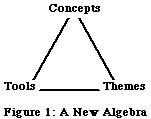## II. Twenty-Four Activities

As an example of this approach, we will summarize a group of activities that explore the theme of area. We hope to show the extraordinary wealth of algebraic experiences that result from investigations of this theme with the help of the following math tools: graph paper, function diagrams, geoboards, the Lab Gear (an algebra manipulative), scientific calculators, and electronic graphing utilities. Many algebra skills and concepts are illuminated by this work. We will pay special attention to the concept of square root, which is usually mistaught in the traditional course.

The activities include some classic problems from the problem-solving movement, and some original ones that have been successful in our classes. However what makes the power of this approach is not the individual activities, but their systematic and coordinated use to pursue algebraic concepts, and the synergism of their interactions. This contextual and tool-based approach to Algebra provides an alternative to the piecemeal implementation or outright non-implementation of the NCTM Standards which might become the norm in the absence of a new paradigm.

As you read this, we suggest you work the problems. While at an entry level they are accessible to all students, many have enough depth to be of genuine interest to math teachers -- unlike much of the traditional Algebra 1 curriculum which many teachers view as necessary, but boring. It is this combination of access, motivation, and challenge that makes these problems interesting to a broad cross-section of students, and insures that they are well suited for cooperative learning and heterogeneous classes. As you work, note the variety of the activities, which range from visual, to numerical, to symbolic; from open-ended to narrowly focused; and from exploratory to explanatory. Contrast this with the monotony of the traditional course. Varying the style of the activities makes it possible to reach students with different learning styles, to avoid the deadening routine of unmotivated drill, and to attain a richness in mathematical content which would be impossible in the traditional format.

What follows is an overview. These activities do not constitute a "unit" that can be taught in a limited time, nor is the order in which they are presented necessarily the best. They are intended to be spread through the school year, and supported by other work. All the activities have been used successfully in relatively heterogeneous Algebra 1 classes.

### Area on Graph Paper

A polyomino is a figure consisting of unit squares, joined to each other side-to-side. Polyominoes can be discovered with the help of manipulatives (cubes or tiles), or (not as effectively) simply by drawing them on graph paper. Thinking about polyominoes leads to many questions in the fields of combinatorial geometry and recreational mathematics. Some of these can be touched on in an Algebra 1 course, as a way to provide connections with these other parts of mathematics, but pursuing them in depth would take us too far afield in this paper.

After finding the polyominoes up to area 5 to develop a feel for what they are, the key problems that will concern us are:

1. For polyominoes of a given area, what perimeters are possible?
2. More specifically, for polyominoes of a given area, what is the longest possible perimeter? the shortest?

Investigating these questions (with some teacher guidance if necessary) leads students to build tables, look for patterns, and graph data (both linear and non-linear), activities recommended in the Standards. The table that is the result of this investigation is in Figure 2. (See also Picciotto, 1986.)

 Area Shortest Perimeter Longest Perimeter 1 4 4 2 6 6 3 8 8 4 8 10 5 10 12 6 10 14 7 12 16 8 12 18 9 12 20 10 14 22 11 14 24 12 14 26 13 16 28 14 16 30 15 16 32 16 16 34 17 18 36 18 18 38 19 18 40 20 18 42 21 20 44 22 20 46 23 20 48 24 20 50

Figure 2: Polyomino Area / Perimeter Table

A generalization in words and in symbolic notation is readily accessible to almost all students, in answer to the question about the longest perimeter. The longest perimeter is equal to twice the area, plus two. Or: P = 2A + 2. This formula can be justified by referring to the table of values obtained empirically, or more meaningfully by a geometric argument based on the shape of polyominoes with maximum perimeter.

The longest perimeter, as a function of area, can also be represented by a function diagram. (See Figure 3.)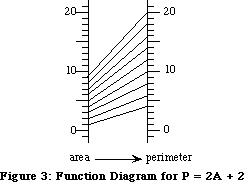The function diagram is a tool for thinking about functions which complements Cartesian graphing, by emphasizing different features of functions. For example, domain and range, the definition of function, inverse function, and rate of change, are easier to understand with the help of this tool than with Cartesian graphs (Arcavi, 1989). In the example above, it is clear that whenever x increases by 1, y increases by 2.

The shortest perimeter is a substantially more difficult question, which will probably not be fully solved in the first few days of school. Nevertheless, it is interesting to struggle with it, and most classes will discover a pattern there.

Finally, the Cartesian graphs for the longest and shortest perimeters (see Figure 4) can provide the basis for an interesting discussion of the findings of this investigation. We will return to this later.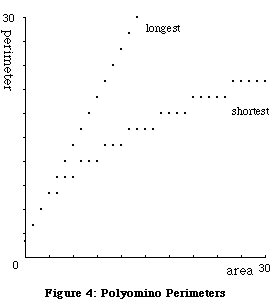### Area of a Rectangle

The rest of the lessons assume familiarity with the idea that the area of a rectangle is the product of its length and width. Students probably need to review this, preferably with the help of manipulatives (cubes or tiles) and/or graph paper. A good way to achieve this review is with the following problems:

1. How many different rectangles of area 24 can you make such that their lengths and widths are whole numbers?
2. Answer the same questions for whole-number areas from 1 to 36.

### Area on Geoboards

Another grid tool is the geoboard, a square lattice of pegs on which students create figures with the help of rubber bands. Work started on geoboards can be continued on dot paper, as the need for the manipulative decreases. Many interesting geometric questions can be investigated with the help of geoboards. We will concentrate on the following strand, which leads into algebra-rich territory:

1. Find geoboard shapes of area 10. (Almost any whole number would provide an equally interesting activity.)

The purpose of this is for students to start looking for shapes on the grid whose sides are not necessarily horizontal or vertical. It is also an opportunity to learn about Cartesian coordinates as a way to communicate about geoboard pegs. Some sample answers can be seen in Figure 5.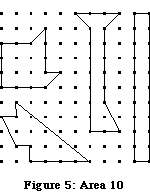1. Find the area of geoboard triangles with one horizontal AND one vertical side.

This helps students use what they know about the area of a rectangle to find the area of a certain type of triangle. It is essential for the teacher to refrain from teaching any area formulas in this unit, since the mere exposure to formulas they have not themselves constructed often numbs students, puts them in a mechanical mode, and interferes with their ability to think. All area-finding techniques should originate among the students. (See Figure 6 for triangles of the types discussed in Problems 6-8.)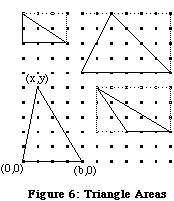1. Find the area of geoboard triangles with one horizontal OR one vertical side.

This helps students develop new techniques for finding geoboard areas, including especially addition, subtraction, and division by two. Too many students have the pseudo-understanding that "area is when you multiply". This activity is a good chance to clarify the meaning of area, while discussing operations in a concrete context.

1. Find the area of geoboard triangles with vertices at (0,0), (b,0),(x,y).

Again, a full investigation of this leads to interesting tables, patterns, and functions, and to the use of variables. In particular, it is interesting to discover that the area is a function of b and y, but not of x.

Problems like #2 and #8 give students opportunities to seek patterns in numerical data. It is useful at this level to analyze data of a mathematical nature, which is easier to work with. (This is not to deny the importance of dealing with "real world" data, which should also be done.)

1. Find geoboard squares of as many different sizes as you can.

33 different squares can be found on an 11 by 11 geoboard. It is visually challenging for students to find them all, particularly the ones whose sides are not horizontal or vertical. Figure 7 shows a few examples. An interesting discussion of slope, and of the slope of perpendicular lines, can be generated by this research, but we will not get into it here. Another interesting tangent is the exploration of the number of squares on a geoboard of dimensions n by n, as a function of n.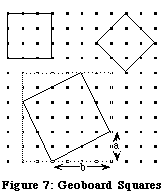1. Find the areas of geoboard squares.

With horizontal-vertical squares, this leads to a discussion of perfect squares. With other squares, another interesting pattern, more complex, can be found. A subtraction-based strategy is to embed the square in a larger horizontal-vertical square, as shown in Figure 7, and subtract the extra triangles. (An interesting investigation at this point is to use this technique to find the area of squares built on the sides of right triangles, which of course leads to the discovery of the Pythagorean theorem.)

After working many specific examples, a strategy can be developed to find the areas efficiently. Students may even find a formula: A = (a+b)2-2ab, where a and b are defined as in Figure 7, and A is the area. Simplifying is not necessary at this stage, but if students know how to do it, they will find an interesting result.

1. Find the sides of squares, given their area.

This is an opportunity to define the square root of a number, both in the context of perfect squares (horizontal-vertical squares), and in general. Sides of squares of known area can be measured on centimeter dot paper. Calculators can be used to get more exact values.

1. Find the distance between any two geoboard pegs.

Students at this level don't know the distance formula and they may not even know the Pythagorean Theorem or think of using it if they do. However all the work with area and Problem 11 have prepared the students for the following method: construct a square, one side of which is the segment connecting the pegs; find its area; take the square root.

1. Use Figure 8 and the concept of area of a square to explain why a calculator gives the same value for 2·SQRT(5) and SQRT(20).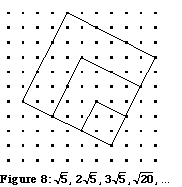1. A similar question could be asked about 3·SQRT(5) and the square root of another number. What is the number? Explain.

After discussing these problems, students can be asked to generalize about n·SQRT(5), where n is a natural number.

### Area with the Lab Gear

The Lab Gear is a manipulative system that can be used to model many of the concepts and techniques of algebra (Picciotto, 1990). Part of the model rests on "the area model of multiplication", in other words the fact that the area of a rectangle is equal to the product of its length and width. Figure 9 shows some of the components of the Lab Gear, three blocks representing numbers, and two representing variables.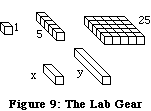Once the x and y blocks have been defined, it is possible to identify the rest of the blocks. For example, Figure 10 shows 5x. The value of this block can be seen by counting, or by placing it in the corner piece, where the dimensions of the rectangle can readily be found.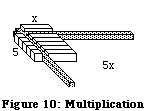By this method, the rest of the blocks can be identified. (See Figure 11.)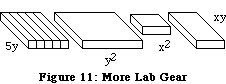1. Show 6x2 with the Lab Gear. Rearrange the blocks into a rectangle. For this rectangle, write the equation "length times width equals area". Rearrange the blocks into another rectangle, and write an equation for it too.

This activity can even be taken to three dimensions, in the context of volume of a box.

1. Repeat the previous problem with 2x2+4x, then with 2x2+4x+2.

Work with manipulatives, by giving students "hands on" experience with variables, can help them avoid some common mistakes. For example a beginner's confusion between 2x, 2 + x, and x2 is quickly dispelled. These quantities all appear in Figure 12: it is clear they do not even remotely resemble each other.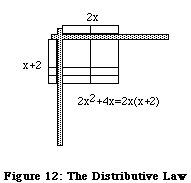The purpose of the above activity (and others like it) is for students to develop a visual understanding of the distributive rule. The problem was equivalent to factoring the expression. Starting with this form of factoring helps students develop a feel for the distributive law, which is then easily introduced, initially in the form: "multiply every block along the left by every block across the top -- make sure you get a rectangle (or a square)."

The Standards encourage us to put less emphasis on factoring. This is absolutely correct in that factoring is not a very important skill, either in mathematics or its applications. However it is an important concept, and it would be a mistake to eliminate factoring from the curriculum. Developing skills in factoring complicated expressions is a waste of time, but a student who cannot factor any algebraic expression does not fully understand multiplication or the distributive law.

Factoring and distributing with manipulatives can help students avoid another common mistake: "distributing the square". (x+5)2 can easily be built with the Lab Gear, and it is clearly not equal to x2+25. This representation can be included in a hands-on development of completing the square. In fact, the identity for (a+b)2 can be discovered with the Lab Gear, and one can use that information to simplify the expression found above for the area of a square while working on the geoboard.

Mentally juxtaposing the Lab Gear with the geoboard leads to the idea of a new tool: "rad gear", or Lab Gear-like blocks in sizes suggested by the geoboard squares. For example, a set of blocks can be made by pasting centimeter dot paper onto cardboard, and cutting it according to the pattern shown in Figure 13.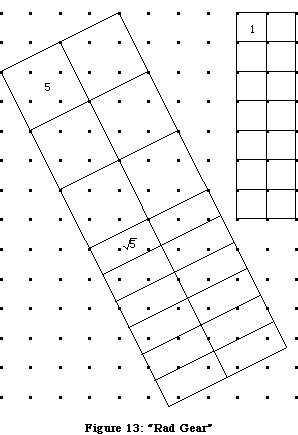1. Make a rectangle in the corner piece, using six "rad gear" blocks of area 5.
1. Write an equation in the form "length times width equals area".
2. Check the multiplication with a calculator.
2. Repeat, using four SQRT(5)-blocks and two 5-blocks.

These kinds of problems lay the groundwork for a more solid understanding of what happens when manipulating radicals. The goal is not accuracy in the obsolete skill of complex simplifications that involve many radicals. Rather, it is to better understand the square root, a fundamental operation.

### "Real World" Area Problems

The examples we have looked at so far were generated by the interaction of the theme of area with various classroom tools. Another direction we can go in is to look at area in the context of so-called "real world" problems.

1. You have 28 feet of fencing for a rectangular pen. How are the length, width, and area of the pen related?

Again, students can build tables, graphs, and function diagrams based on their empirical investigation. Again patterns emerge, and can be expressed algebraically.

A connection can be made with the Lab Gear by calling the length and width x and y. Then you have 2x+2y=28, and the blocks allow a manipulative solution to the problem of expressing y in terms of x. (See Figure 14. A Lab Gear convention is that any block placed "upstairs" -- on top of another block -- is preceded by a minus sign. The uncovered part of the downstairs block represents "what's left" after a subtraction. The vertical line represents the equal sign.)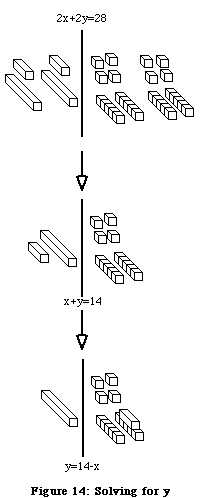We find that y = 14-x, so the area can be expressed in terms of x: A = x(14-x)

Once functions are expressed in terms of one variable, x, they can be entered into the graphing calculator. Having done the graphing by hand in simple cases allows students to use electronic graphing with more understanding. (It is neither necessary nor desirable to see a graphing utility as a "black box".) See Figure 15 for the graphs as they appear in an electronic grapher. The graphs can be used to help answer the following problems:

1. What dimensions give the maximum area if the perimeter of a rectangle is given?

Note that we preceded this question with the general question of the relationship among the three variables, before focusing on the maximizing problem. This gives students a chance to decide for themselves which graph to use to answer the question.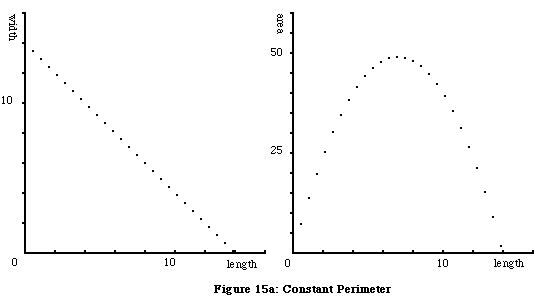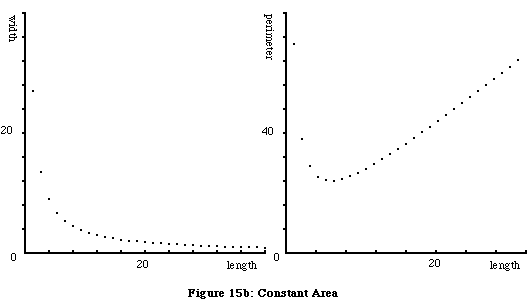1. You can afford 36 square feet of Astroturf for a rectangular pen. How are the length, width, and perimeter of the pen related?

Because by now they have had much practice with functional relationships in several representations, students can derive the function expressing perimeter in terms of one of the sides (P = 2x + 72/x). A generalized understanding of the behavior of functions of this type is "too difficult for Algebra 1". However, after having worked with simpler examples, and with the help of an electronic grapher, it is perfectly legitimate to stretch the students with the following two problems.

1. What dimensions give the shortest perimeter for a rectangle if the area is given?
2. Is there a rectangle with perimeter 28 and area 36?

### Graphing Square Roots

In looking back over the problems discussed so far, one can see that the use of a variety of math tools empowers students to develop more than one representation for algebra concepts. In particular, we have looked at the concept of square roots with the help of geoboards, the Lab Gear model, and calculators. How about trying to use the graphing calculator to approach the same concept in a fourth way?

1. What numbers have a square root that is
1. equal to the number?
2. less than the number?
3. greater than the number?

It's a good idea to start out by exploring this by trial and error on a scientific calculator. However, students will gain more insight by graphing the functions y = √x and y = x. (See Figure 16.)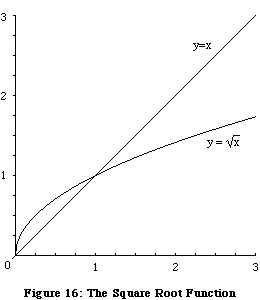### An Extension

An observant reader with photographic memory might notice that the shape of the square root graph is somewhat reminiscent of the graph of minimum perimeter for a given polyomino area. The two graphs are shown on the same axes in Figure 17.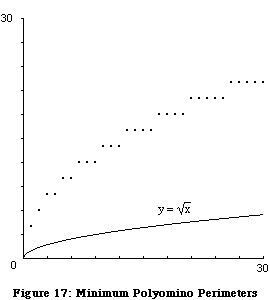Fiddling with the graphs by changing the parameters may help us get a better fit. (See Figure 18.) Notice that comparing with 4 graphically is a helpful reminder of the ideas we had already seen on the geoboards and with the "radical gear".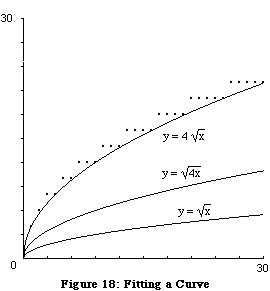We have not exhausted this problem, of course. We could improve the fit by shifting the curve up by one unit, or better yet, by using the greatest integer function. Finally, we could discuss the reason for this fit by connecting what we learned in the minimum perimeter problem (in the continuous case of the rectangle with given area) with the data that originated in the minimum perimeter problem (in the discrete case of a polyomino of given area). How far to pursue this with your students will depend on your class and your judgment.

## III. Tools, Themes, Concepts

### Tools

While working on activities, we used, or could have used, the following manipulative, electronic, and traditional math tools: grid tools (cubes or tiles, graph paper, geoboards, dot paper); visualization tools (function diagrams, the Lab Gear, Cartesian graphs); computational tools (calculators, graphers); and paper-and-pencil tools (tables of values, symbol manipulation).

We see four main advantages to a tool-based approach:

Access: By providing immediate feedback, these tools make it possible for all students to get involved with significant mathematical concepts: data analysis; using tables, looking for patterns; generalizing and using variables; linear, quadratic, and rational functions; squares, square roots and operations with radicals; the distributive law and factoring; optimization and equation-solving; not to mention connections with many other topics.

Discourse: The tools also facilitate the transition from a traditional class format into one where discovery learning, problem solving, and cooperative work are the norm. They are not only objects to think with, but also objects-to-talk-about. Instead of the teacher's authority being the sole arbiter of correctness, tools make it possible for students to use reasoning and discussion about a concrete reference as a way to judge the validity of mathematical statements.

Independence: As students work with tools over time and develop more and more understanding of the concepts of algebra, they have less and less need of certain tools (such as manipulatives or function diagrams), which have merely served as a bridge to understanding abstract ideas. On the other hand, they become more sophisticated users of other tools, (such as calculators and electronic graphers), which will remain useful throughout their mathematical careers. In both cases, the students are more self-reliant, and therefore more self-confident.

Multiple Representations: There is a synergy in the interaction of math tools. A student who has thought about square roots in a multidimensional way, with the help of geoboards, dot paper, radical gear, calculators, and graphing calculators, has much more depth of understanding than one who has only practiced disembodied operations with radicals, particularly if the relationships among the representations have been made explicit.

### Themes

The twenty-four activities were all inspired by the theme of area. The various tools, themes and concepts we touched on are all inter-related, as can be seen on the map of these connections (Figure 19). Naturally, we were not able to address everything shown on the map in this paper, but it is not unrealistic to expect many of these topics to be among the ones addressed in a one-year course. Note that the numerous connections between algebra topics, and between them and geometric topics are revealed by the thematic approach, while it is obscured in the traditional curriculum which assumes an arbitrary sequence within algebra, and fails to make the geometric connections. (The corresponding map for the traditional algebra course can be seen in Figure 20.)

As shown above, geometric themes such as area and distance are a gold mine of exciting work in Algebra. However, since an introductory Algebra course needs to include topics such as exponential functions, laws of exponents, equations and inequalities, and so on, other themes will be needed. Here are some examples of themes which we have found to be rich with mathematical content at the appropriate level: motion; optimization; growth and change; satisfying constraints; making comparisons. Well-chosen themes offer:

Connections within algebra and to other branches of mathematics.

Motivation for specific topics and for learning algebra in general.

Applications to other fields.

Spiraling, since a core idea can be previewed, then studied, and later on reviewed, each time in the context of a different theme.

### Concepts

While symbol manipulation is a useful tool, accurate and/or speedy manipulation is no longer defensible as a central goal of the new algebra. Instead the goal should be understanding of concepts. Tools and themes are the means, not the end: their purpose is to help create a course where students can learn algebra concepts such as function, numbers, variables, operations, equations, and more generally mathematical structure. Tools and themes create an environment where students are empowered and motivated, where problem solving, discovery and cooperative learning can thrive, and where skills can develop naturally and in context.

While they are necessary, even well-chosen tools and themes are not sufficient to guarantee that students will generalize and transfer their understanding from one context to another. Essentially, this will come from our students learning how to think mathematically, which is best achieved through group work, teacher-led discussion, and writing, and best monitored through a range of assessment techniques such as reports, projects, portfolios, and tests. These changes, which are well presented in the NCTM Standards (1989, 1991), must be made in conjunction with the implementation of the contextual tool-based approach.

As students' understanding of algebra concepts deepens, they are gaining symbol sense: an appreciation for the power of symbolic thinking, an understanding of when and how to apply it, and a feel for mathematical structure. Symbol sense is a level of mathematical literacy beyond number sense, which it subsumes. It is the true prerequisite for further work in math and science, and the real purpose of a new Algebra course.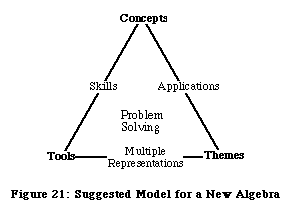## Bibliography

1. Arcavi, Abraham and Nachmias, Rafi (1989) "Re-exploring familiar concepts with a new representation." Proceedings of the 13th International Conference on the Psychology of Mathematics Education., 3, 174-181.
2. College Entrance Examination Board (CEEB). (1990) Changing the Odds. New York: CEEB.
3. National Council of Teachers of Mathematics (NCTM). (1989) Curriculum and Evaluation Standards for School Mathematics. Reston, VA: NCTM.
4. National Council of Teachers of Mathematics (NCTM). (1991) Professional Standards for Teaching Mathematics. Reston, VA: NCTM.
5. Papert, Seymour. (1980) Mindstorms: Children, Computers, and Powerful Ideas. New York: Basic Books.
6. Picciotto, Henri. (1990) The Algebra Lab. Sunnyvale, CA: Creative Publications.
7. Picciotto, Henri. (1986) Polyomino Lessons. Sunnyvale, CA: Creative Publications.Related pages on this site:
For a Tool-Based Pedagogy
Algebra: Themes, Tools, Concepts
The Pythagorean Geoboard
Math: Visual and Interactive